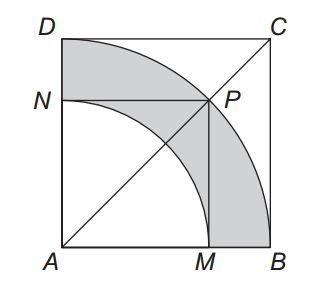# Irrationally simple

Geometry Level 3In the figure above, $ABCD$ and $AMPN$ are squares and $BD$ and $MN$ are arcs of circles with center $A$.

What is the ratio of the area of the grey region and the square $ABCD$?

×

Problem Loading...

Note Loading...

Set Loading...